Search by Topic

Resources tagged with Polyhedra similar to Rectangular Pyramids:

Filter by: Content type:
Age range:
Challenge level:

There are 22 results

Broad Topics > 3D Geometry, Shape and Space > Polyhedra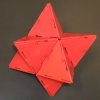Investigating Solids with Face-transitivity

Age 14 to 18

In this article, we look at solids constructed using symmetries of their faces.Dodecawhat

Age 14 to 16 Challenge Level:

Follow instructions to fold sheets of A4 paper into pentagons and assemble them to form a dodecahedron. Calculate the error in the angle of the not perfectly regular pentagons you make.Lighting up Time

Age 7 to 14 Challenge Level:

A very mathematical light - what can you see?Three Cubes

Age 14 to 16 Challenge Level:

Can you work out the dimensions of the three cubes?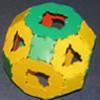Platonic and Archimedean Solids

Age 7 to 16 Challenge Level:

In a recent workshop, students made these solids. Can you think of reasons why I might have grouped the solids in the way I have before taking the pictures?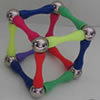Magnetic Personality

Age 7 to 16 Challenge Level:

60 pieces and a challenge. What can you make and how many of the pieces can you use creating skeleton polyhedra?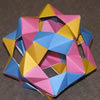Plaited Origami Polyhedra

Age 7 to 16 Challenge Level:

These models have appeared around the Centre for Mathematical Sciences. Perhaps you would like to try to make some similar models of your own.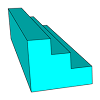Which Solid?

Age 7 to 16 Challenge Level:

This task develops spatial reasoning skills. By framing and asking questions a member of the team has to find out what mathematical object they have chosen.Tetra Square

Age 11 to 14 Challenge Level:

ABCD is a regular tetrahedron and the points P, Q, R and S are the midpoints of the edges AB, BD, CD and CA. Prove that PQRS is a square.Sliced

Age 14 to 16 Challenge Level:

An irregular tetrahedron has two opposite sides the same length a and the line joining their midpoints is perpendicular to these two edges and is of length b. What is the volume of the tetrahedron?Tetrahedra Tester

Age 11 to 14 Challenge Level:

An irregular tetrahedron is composed of four different triangles. Can such a tetrahedron be constructed where the side lengths are 4, 5, 6, 7, 8 and 9 units of length?Proximity

Age 14 to 16 Challenge Level:

We are given a regular icosahedron having three red vertices. Show that it has a vertex that has at least two red neighbours.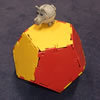Platonic Planet

Age 14 to 16 Challenge Level:

Glarsynost lives on a planet whose shape is that of a perfect regular dodecahedron. Can you describe the shortest journey she can make to ensure that she will see every part of the planet?Classifying Solids Using Angle Deficiency

Age 11 to 16 Challenge Level:

Toni Beardon has chosen this article introducing a rich area for practical exploration and discovery in 3D geometryTet-trouble

Age 14 to 16 Challenge Level:

Is it possible to have a tetrahedron whose six edges have lengths 10, 20, 30, 40, 50 and 60 units?Icosian Game

Age 11 to 14 Challenge Level:

This problem is about investigating whether it is possible to start at one vertex of a platonic solid and visit every other vertex once only returning to the vertex you started at.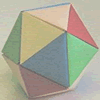Paper Folding - Models of the Platonic Solids

Age 7 to 16

A description of how to make the five Platonic solids out of paper.Triangles to Tetrahedra

Age 11 to 14 Challenge Level:

Imagine you have an unlimited number of four types of triangle. How many different tetrahedra can you make?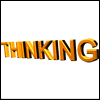Thinking 3D

Age 7 to 14

How can we as teachers begin to introduce 3D ideas to young children? Where do they start? How can we lay the foundations for a later enthusiasm for working in three dimensions?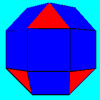Rhombicubocts

Age 11 to 14 Challenge Level:

Each of these solids is made up with 3 squares and a triangle around each vertex. Each has a total of 18 square faces and 8 faces that are equilateral triangles. How many faces, edges and vertices. . . .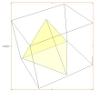A Mean Tetrahedron

Age 11 to 14 Challenge Level:

Can you number the vertices, edges and faces of a tetrahedron so that the number on each edge is the mean of the numbers on the adjacent vertices and the mean of the numbers on the adjacent faces?Which Solids Can We Make?

Age 11 to 14 Challenge Level:

Interior angles can help us to work out which polygons will tessellate. Can we use similar ideas to predict which polygons combine to create semi-regular solids?